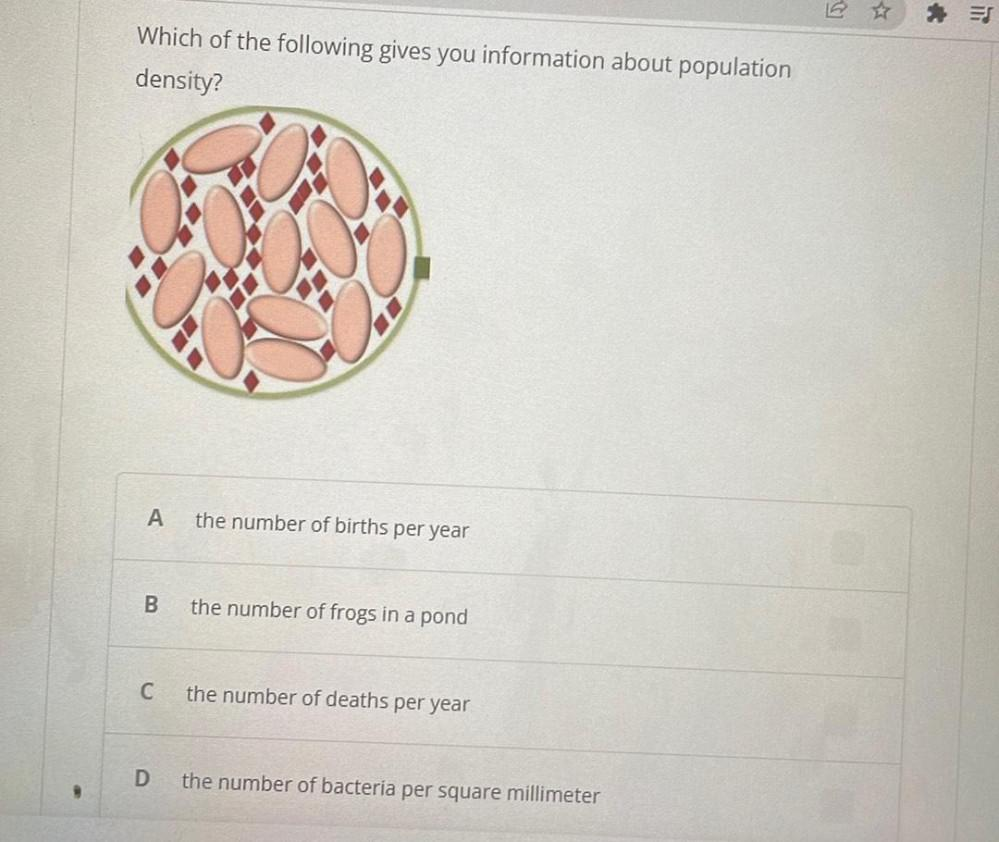Question:

# Which of the following gives you information about population density? <IMAGE> A. The number of births per year B. The number of frogs in a pond C. The number of deaths per year D. The number of bacteWhich of the following gives you information about population density? <IMAGE> A. The number of births per year B. The number of frogs in a pond C. The number of deaths per year D. The number of bacteria per square millimeter